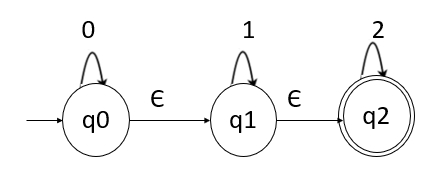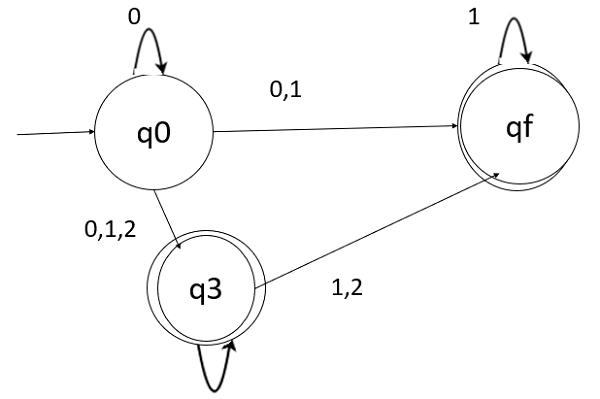# How to convert NFA with epsilon to without epsilon?

In this method, we try to remove all the ε-transitions from the given Non-deterministic finite automata (NFA) −

The method is mentioned below stepwise −

• Step 1 − Find out all the ε-transitions from each state from Q. That will be called as ε-closure(qi) where, qi ∈Q.
• Step 2 − Then, 𝛿1 transitions can be obtained. The 𝛿1 transitions means an ε-closure on 𝛿 moves.
• Step 3 − Step 2 is repeated for each input symbol and for each state of given NFA.
• Step 4 − By using the resultant status, the transition table for equivalent NFA without ε can be built.

NFA with ε to without ε is as follows −

δ1(q,a) = ∈ - closure (δ (δ∈^(q,∈𝛜),a)) where, δ^(q,∈𝛜) = ∈ - closure(q)

## Example

Convert the given NFA with epsilon to NFA without epsilon.## Solution

We will first obtain ε-closure of each state i.e., we will find ε-reachable states from the current state.

Hence,

• ε-closure(q0) = {q0,q1,q2}
• ε-closure(q1) = {q1,q2}
• ε-closure(q2) = {q2}

ε-closure(q0) means with null input (no input symbol) we can reach q0, q1, q2.

In a similar manner for q1 and q2 ε-closure are obtained.

Now we will obtain 𝛿1 transitions for each state on each input symbol as shown below −

δ'(q0, 0) = ε-closure(δ(δ^(q0, ε),0))
= ε-closure(δ(ε-closure(q0),0))
= ε-closure(δ(q0,q1,q2), 0))
= ε-closure(δ(q0, 0) ∪ δ(q1, 0) U δ(q2, 0) )
= ε-closure(q0 U Φ ∪ Φ)
= ε-closure(q0)
= {q0,q1, q2}

δ'(q0, 1) = ε-closure(δ(δ^(q0, ε),1))
= ε-closure(δ(q0,q1,q2), 1))
= ε-closure(δ(q0, 1) ∪ δ(q1, 1) U δ(q2, 1) )
= ε-closure(Φ ∪q1 U Φ)
= ε-closure(q1)
= {q1, q2}

δ'(q0, 2) = ε-closure(δ(δ^(q0, ε),2))
= ε-closure(δ(q0,q1,q2), 2))
= ε-closure(δ(q0, 2) ∪ δ(q1, 2) U δ(q2, 2) )
= ε-closure(Φ U ΦU q2)
= ε-closure(q2)
= {q2}

δ'(q1, 0) = ε-closure(δ(δ^(q1, ε),0))
= ε-closure(δ(q1,q2), 0))
= ε-closure(δ(q1, 0) U δ(q2, 0) )
= ε-closure(Φ ∪ Φ)
= ε-closure(Φ)
= Φ

δ'(q1,1) = ε-closure(δ(δ^(q1, ε),1))
= ε-closure(δ(q1,q2), 1))
= ε-closure(δ(q1, 1) U δ(q2, 1) )
= ε-closure(q1 ∪ Φ)
= ε-closure(q1)
= {q1,q2}

δ'(q1, 2) = ε-closure(δ(δ^(q1, ε),2))
= ε-closure(δ(q1,q2), 2))
= ε-closure(δ(q1, 2) U δ(q2, 2) )
= ε-closure(Φ ∪ q2)
= ε-closure(q2)
= {q2}

δ'(q2, 0) = ε-closure(δ(δ^(q2, ε),0))
= ε-closure(δ(q2), 0))
= ε-closure(δ(q2, 0))
= ε-closure(Φ)
= Φ

δ'(q2, 1) = ε-closure(δ(δ^(q2, ε),1))
= ε-closure(δ(q2), 1)
= ε-closure(δ(q2, 1))
= ε-closure(Φ)
= Φ

δ'(q2, 2) = ε-closure(δ(δ^(q2, ε),))
= ε-closure(δ(q2), 2))
= ε-closure(δ(q2, 2))
= ε-closure(q2)
= {q2}

Now, we will summarize all the computed δ' transitions as given below −

δ'(q0,0)={q0,q1,q2}
δ'(q0,1)={q1,q2}
δ'(q0,2)={q2}

δ'(q1,0)= { Φ }
δ'(q1,1)={q1,q2}
δ'(q1,2)={q2}

δ'(q2,0)={ Φ }
δ'(q2,1)={ Φ }
δ'(q2,2)={q2}

The transition table is given below −

States\inputs012
q0{q0,q1,q2}{q1,q2}{q2}
q1Φ{q1,q2}{q2}
q2ΦΦ{q2}

## NFA without epsilon

The NFA without epsilon is given below −Here, q0, q1, q2 are final states because ε-closure(q0), ε-closure(q1) and ε-closure(q2) contain a final state q2.